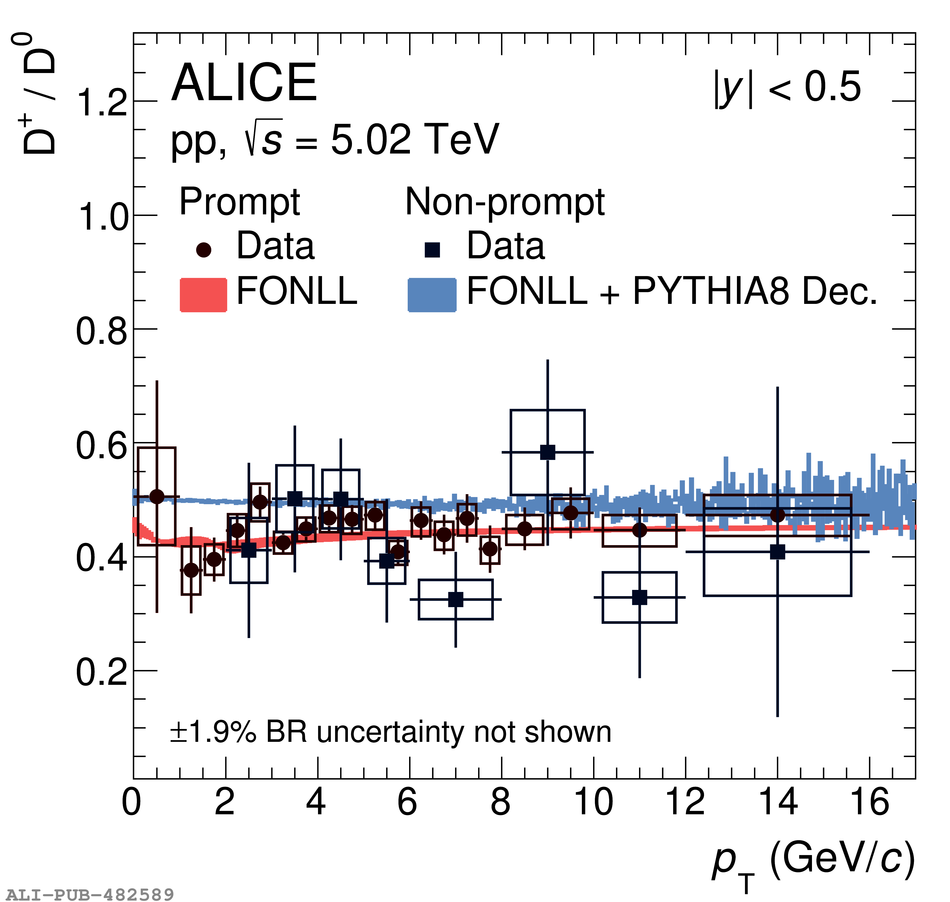# Figure 9

 < p>Ratios between the $\pt$-differential production cross sections of $\Dplus$ and $\Dzero$ mesons (left panel) and between the one of $\Ds$ mesons and the sum of the $\Dzero$- and $\Dplus$-meson cross sections (right panel) compared with predictions obtained with FONLL calculations~ and PYTHIA~8~ for the $\bhad\to\mathrm{D+X}$ decay kinematics. For the non-prompt $\Ds/(\Dzero+\Dplus)$ ratio, the predictions for the $\Ds$ from $\Bs$ and from non-strange B meson decays are also displayed separately.< /p>